## Abstract

Karanja is a medium sized evergreen tree which has minor economic importance in India. The nonedible seed kernel contains 27–30% oil that is used for biodiesel production, leaving the remaining nonedible seed cake as a waste product. The aim of the present work was to obtain kinetic parameters in relation to technological parameters in nonedible seed cake biomass pyrolysis conversion process to bio-oil and biochar. Effects of heating rate on karanja seed cake slow pyrolysis behavior and kinetic parameters were investigated at heating rates of 5, 10, and 20°C/min using thermogravimetric analysis (TGA). Thermogravimetric experiments showed the onset and offset temperatures of the devolatilization step shifted toward the high-temperature range, and the activation energy values increased with increasing heating rate. In the present study, isoconversional method was applied for the pyrolysis of karanja seed cake biomass by TGA and the activation energies (118–124 kJ/mol) and the pre-exponential factors obtained using progressive conversion. Proximate–ultimate analyses, energy value, surface structure, and Fourier transform infrared spectra of the biomass processed under conditions were reported. The pyrolysis resulted in upgradation of the energy value of seed cake biomass from 18.1 to 24.5 MJ/kg; importantly with high carbon and low oxygen contents. The approach represents a novel method for the upgrading of karanja seed cake that has significant commercial potential.

## Introduction

With the growing concerns about fossil fuel exhaustion and environmental problems such as global warming, efforts are underway to decrease CO2 emission rates and to develop alternative energies which substitute for limited fossil fuels. Biodegradable nontoxic biomass having low emission profiles compared to petroleum-based fuels (petrol and diesel) is now widely recognizing as a potential energy source.

Biomass pyrolysis is a thermal decomposition of biomass taking place in the absence of oxidizing agent with products biochar, bio-oil, and gases such as carbon dioxide, carbon monoxide, hydrogen, and methane. Pyrolysis is a promising technology for the production of bio-oil and biochar. Bio-oil, also known as pyrolytic oil, can be upgraded to light hydrocarbons which contain low levels of aromatics with the absence of sulfur compared to petroleum-based fuels. Biochar, on the other hand, is an ecofriendly carbon-rich product from the pyrolysis of renewable feedstocks like nonedible seed cakes after the extraction of oil and has been widely used in agriculture as a soil amendment and for improving soil fertility due to its carbon sequestration ability . Biochar is also reported to promote nitrogen fixation thereby decreasing the emission of N2O  and other greenhouse gases from agricultural soils [3, 4]. In addition, biochar has been used as an adsorbent in the removal of arsenic , cadmium , and chromium from aqueous solutions.

Pyrolysis process occurs with high heat flux to the biomass with a corresponding high heating rate of the biomass particle and heat transfer to the biomass in a very short time period. Applying heat to biomass at high rate will result into smaller fragments (volatiles) due to the cleavage of cellulose, hemicellulose, protein, and lignin, and other constituents of biomass, unstable above 400°C due to the presence of oxygen in the fragments. Hence, very short residence times are required in the thermal treatment step followed by immediate quenching to impede secondary chemical change of unstable volatiles. Pyrolysis with a short residence time and optimum temperature of exposure with immediate cooling of the vapors can be achieved by taking into account the appropriate size of the reactor and heating rate in terms of heat flux. Heat transfer rate to biomass particles and residence time of vapors in the reactor have a strong influence on the nature and distribution of pyrolysis reaction products. Therefore, the first and foremost consideration for designing biomass pyrolysis reactor (residence time, size, and material of construction) is to draw the clear picture of thermal behavior of the feedstock biomass with the application of heat at different heating rate which corresponds to heat transfer rate to biomass particle in order to estimate the amount of energy to be supplied for pyrolysis.

Various biomass feedstocks show different thermal behavior profiles mainly due to the variation in the composition of biomass constituents. For instance, Parthasarathy and Sheeba  have investigated the thermal behavior of bagasse, coir pith, groundnut shell, and casuarinas leaves, which exhibited different degradation temperatures of individual biomass components (hemicellulose, cellulose, and lignin).

Vhathvarothai et al.  have studied the copyrolysis of cypress wood chips, macadamia nut shells biomass, and coal using thermogravimetric technique and Kissingers corrected kinetic equation for kinetic parameters determination and concluding that the activation energy of coal is greater than that of both types of biomass.

Pyrolysis kinetics of raw and hydrothermally carbonized loblolly pine lignocellulosic biomass, performed in a thermogravimetric analyzer, was carried out by Yan et al. . The study revealed that pyrolysis of hydrothermally carbonized biomass progressed less aggressively than that of raw biomass which also showed that after an initial significant decomposition, the pyrolysis reactions continued at much slower rates with temperature.

The current research interest is to create a platform for deoiled karanja seed cake biomass in biorefinery by producing energy and value-added products. The study is aimed at thermal behavior of the biomass under pyrolysis conditions which can be used in the design and development of an effective pyrolysis process. Recent research  has indicated the potential of bio-oil and biochar obtained from pyrolysis of the seed cake biomass in areas such as energy production and the production of activated carbon.

Pongamia pinnata or Pongamia glabra known as the karanja tree in India is an evergreen tree of south and Southeast Asia. The Indian Government has initiated karanja tree plantation project in the year 2003 since then 20 million trees have been planted resulting into an annual production of 800 metric tons of karanja seeds in the year 2008 and was increased to 2,00,000 metric tons by 2011 . National oilseeds and vegetable oils Development Board, Ministry of Agriculture, Government of India (2008) has showed the statistics of karanja seed kernals production per one hectare in the range 1958–4405.5 kg kernals/hectare. Nonedible seed oils (30% of entire seed weight) have been successfully processed for biodiesel production (transesterification), the remaining 70% of seed material and deoiled seed cake can be processed by a variety of treatment methods (hydrolysis and fermentation, pyrolysis, and gasification) for energy and chemicals production, which gives an added economic value to the seed cake biorefinery .

The aim of the present work was to study the thermal behavior and pyrolysis kinetics of the nonedible karanja seed cake with different temperature programs using thermogravimetric technique. Characteristic properties including proximate–ultimate analyses, surface structure, surface active functional groups, and higher heating value (HHV) of the raw and thermally treated biomass in a fixed bed pyrolysis reactor are evaluated. The present work is the first report on the use of the nonisothermal methods including model-fitting and model-free methods to calculate the kinetic parameters for the pyrolysis of karanja seed cake.

## Material and Methods

### Materials

Deoiled karanja seed cake used in the present work was obtained from a local producer in Andhra Pradesh, India. The biomass was processed to an average particle size of <1 mm and used as such for thermogravimetric analysis (TGA).

### Thermogravimetric analysis

TGA of karanja seed cake was performed in a Mettler Toledo TGA/SDTA 851e analyzer (Mettler Toledo, Switzerland). The analysis of the samples was performed at three different heating rates, 5, 10, and 20°C/min, using nitrogen with a flow rate of 30 mL/min to a final temperature of 800°C with a sample mass of ~11 ± 0.1 mg. The loss in the weight of the sample was recorded as a function of temperature.

### Kinetic studies

Karanja pyrolysis kinetics was studied using the nonisothermal data obtained from different temperature programs at constant heating rate. International Confederation for Thermal Analysis and Calorimetry (ICTAC) kinetics committee recommendations for performing kinetic computations on thermal analysis data were followed to evaluate all the reliable kinetic parameters. Mathematical analysis to determine the kinetic triplet, activation energy (E), frequency factor (A), and reaction model f(α), was performed by the method of Coats and Redfern (model-fitting approach), and model-free isoconversional Kissinger–Akahira–Sunose (KAS) and Ozawa–Flynn–Wall (OFW) methods .

The conversion of biomass in the pyrolysis process is represented by the following reaction scheme:

 ${\mbox{Biomass}}\rightarrow {\mbox{Biochar}}+{\mbox{Volatile}}$

and the kinetic expression with temperature dependence of the kinetic rate constant, given by Arrhenius equation as:

 ${\frac {d\alpha }{dt}}=k\left(T\right)f\left(\alpha \right)$
(1)

 $k\left(T\right)=A\quad {\mbox{exp}}\left({\frac {-E}{RT}}\right)$
(2)

where f(α) is the reaction model, and it depends on the reaction mechanism, k(T) is the rate constant, A is frequency factor, E the activation energy, T the absolute temperature, t the time, α the degree of conversion, and R the universal gas constant (8.314 J mol−1 K−1). The constant heating rate is defined as:

 ${\mbox{Heating rate}},\quad \beta ={\frac {dT}{dt}}={\mbox{Constant}}$
(3)

 $dt={\frac {dT}{\beta }}$
(4)

Substituting k(T) from equation (2) and dt from equation (4) in equation (1) and after rearrangement, the integration of equation (1) is given as:

 ${\int }_{0}^{\alpha }{\frac {d\alpha }{f(\alpha )}}{\int }_{0}^{T}A\quad {\mbox{exp}}\left({\frac {-E}{RT}}\right){\frac {dT}{\beta }}=$$g\left(\alpha \right)$
(5)

### Model-fitting approach

The solution of equation (5) based on the approximation given in Coats and Redfern  gives rise to the equation form expressed as Coats–Redfern equation, the most frequently used expression to evaluate nonisothermal data to compute kinetic parameters is:

 ${\mbox{ln}}\left({\frac {g(\alpha )}{T^{2}}}\right)={\mbox{ln}}\left({\frac {AR}{\beta E}}\right)\left(1,-\right.$$\left.,{\frac {2RT}{E}}\right)-{\frac {E}{RT}}$
(6)

Conversion (α) in the pyrolysis of karanja seed cake is calculated from TGA data using the equation given below:

 ${\mbox{Conversion}},\quad \alpha ={\frac {m_{i}-m_{\alpha }}{m_{i}-m_{f}}}$
(7)

where mi, mα, and mf are initial, instantaneous, and final mass of the samples, respectively.

The data obtained from TGA were analyzed by different kinetic models representing chemical reaction, nucleation and nuclei growth, surface reaction between both the phases, and diffusion models [13, 15]. The TGA data in the pyrolysis temperature range were analyzed by applying 14 kinetic models given in Table 1 using Coats–Redfern equation. The kinetic model which resulted in a straight line fit with high regression coefficient for ln [g(α)/T2] versus 1/T data was concluded to be the best reaction model representing the experimental data.

Table 1. Kinetic models used in the solid state reactions
Model Mechanism f(α) g(α)
Chemical reaction
1 First order 1 − α [−ln(1 − α)]
2 Second order (1 − α)2 (1 − α)−1 − 1
3 Third order (1 − α)3 [(1 − α)−2 − 1]/2
4 nth order (1 − α)n [(1 − α)1−n − 1]/n − 1
Random nucleation and nuclei growth
5 Two-dimensional 2(1 − α) [−ln(1 − α)]1/2 [−ln(1 − α)]1/2
6 Three-dimensional 3(1 − α) [−ln(1 − α)]2/3 [−ln(1 − α)]1/3
Limiting surface reaction between both phases
7 One dimension 1 α
8 Two dimensions 2(1 − α)1/2 1 − (1 − α)1/2
9 Three dimensions 3(1 − α)2/3 1 − (1 − α)1/3
Diffusion
10 One-way transport 1/2α α2
11 Two-way transport [−ln(1 − α)]−1 α+(1 − α) ln(1 − α)
12 Three-way transport (2/3) (1 − α)2/3/[1 −  (1 − α)1/3] [1 − (1 − α)1/3]2
13 Ginstling–Brounshtein equation (2/3) (1 − α)1/3/[1 −  (1 − α)1/3] 1 − 2α/3 −  (1 − α)2/3
14 Zhuravlev equation (2/3) (1 − α)5/3/[1 −  (1 − α)1/3] [(1 − α)−1/3 − 1]2

### Model-free approach (isoconversional methods)

A number of isoconversional methods are available for evaluating the biomass pyrolysis kinetics. These methods mainly differ in the approximations of the temperature integral in equation (5) and many of them give rise to linear equations. In equation (5) after introducing the following terms:

 ${\mbox{Let}}\quad {\frac {E}{RT}}=p$
 $dp={\frac {-E}{R}}T^{-2}dT$
 ${\mbox{As}}\quad T\rightarrow 0;\quad p\rightarrow \infty \quad {\mbox{and}}\quad T\rightarrow T;\quad p\rightarrow p$

and by substituting E/RT = p on rearrangement the equation is transformed to:

 $g\left(\alpha \right)={\int }_{0}^{\alpha }{\frac {d\alpha }{f(\alpha )}}=$${\frac {A}{\beta }}{\frac {E}{R}}{\int }_{p}^{\infty }p^{-2}{\mbox{exp}}\left(-\right.$$\left.p\right)dp$
(8)

KAS method takes the following approximation for the integral in right-side term of equation (8):

 ${\int }_{p}^{\infty }p^{-2}{\mbox{exp}}\left(-p\right)dp=$$p^{-2}{\mbox{exp}}\left(-p\right)$
(9)

The solution of equation (9) based on the approximation and suitable modification given as equation (10) was used to determine the activation energy and frequency factor.

 $ln{\frac {\beta }{T^{2}}}=ln\left({\frac {AE}{Rg(\alpha )}}\right)-$${\frac {E}{RT}}$
(10)

OFW method applies Doyles approximation  for the integral term on the right side of equation (8), which becomes

 $log\left[{\int }_{p}^{\infty },p^{-2},exp,\left(-p\right),d,p\right]=$$-2.315+0.457p$
(11)

By substituting equation (11) in equation (8) and on rearrangement equation (12) was obtained and was used for calculating the Arrhenius parameters:

 $log\left(\beta \right)=log\left[{\frac {AE}{Rg(\alpha )}}\right]-$$2.315-0.457{\frac {E}{RT}}$
(12)

From KAS method, the plot of ln(β/T2) versus 1/T gives the slope −E/R. From OFW method, activation energy was calculated from the slope (–0.457E/RT) of the straight line obtained by plotting logβ and 1/T data. Frequency factor from the model-free isoconversional methods was determined from the intercept of the plots by making use of the best kinetic model representing the experimental data, f(α), evaluated from Coats–Redfern method.

## Results and Discussion

### TGA of karanja seed cake

The thermal behavior of karanja seed cake in the temperature range 26 ± 2°C to 800°C was studied at three different heating rates. Reduction in the mass of the sample (11 ± 0.1 mg) with temperature at a heating rate 20°C/min is shown in Figure 1. The complete pyrolysis reaction proceeds with an initial dehydration step followed by decomposition of protein, hemicellulose, cellulose, and lignin . In the initial dehydration step, free/bound moisture and volatile extractives of biomass are carried away by the inert gas flow. The dehydration step occurs to a temperature of 200°C. Most of cellulose and hemicellulose decomposes above 200°C  and a vigorous mass loss was observed in the temperature range 250–450°C. Lignin decomposition takes place through a wide temperature range (160–900°C)  because of its recalcitrant structure, and in the present study a very slow mass loss after a temperature of 450°C was attributed to the lignin mass loss.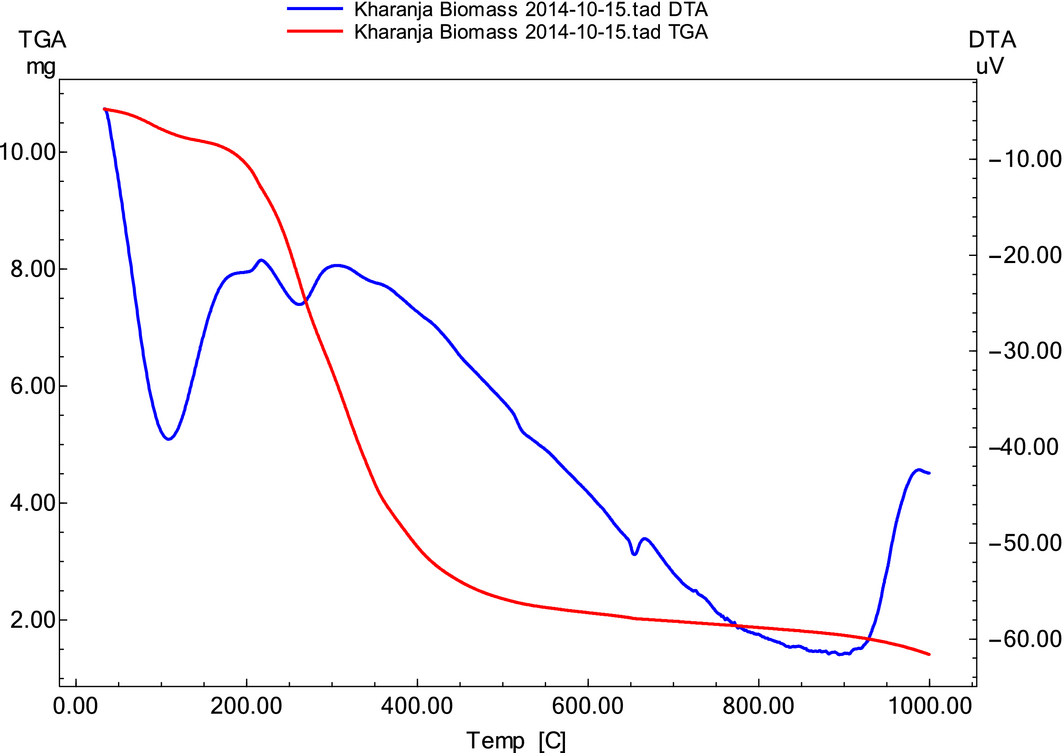Figure 1. Thermogram of karanja seed cake at 20°C/min heating rate.

In the initial dehydration step, a 1.35% weight loss was observed as moisture was removed. In the decomposition of biomass constituents, considered as an active pyrolysis zone, a 73.8% weight loss was observed with the remaining 24.85% representing residual matter. During the pyrolysis, the intermolecular associations and weaker chemical bonds are destroyed. The side aliphatic chains may be broken and some small gaseous molecules are produced at lower temperature. At higher temperatures of decomposition, chemical bonds in lignin are broken and the parent molecular skeletons are destroyed [20, 21].

### Pyrolysis kinetics

Biomass pyrolysis includes complex heat and mass transfer phenomena, therefore only about 11 mg of karanja seed cake biomass samples were used for TGA to minimize/overcome the endothermic and exothermic effects on the sample due to the furnace temperature. The thermogravimetric data were evaluated using 14 different reaction models given in Table 1. The best correlation was obtained with the third-order reaction model [f(α) = (1 − α)3] at three different heating rates and the regression plots according to Coats–Redfern method are shown in Figure 2A.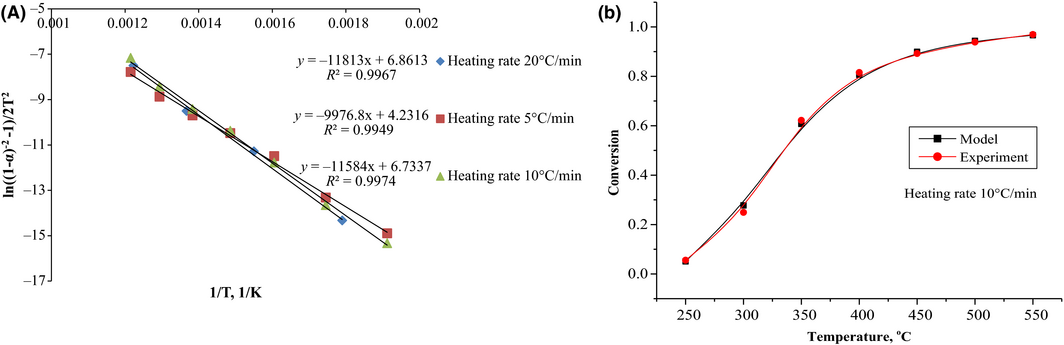Figure 2. Coats–Redfern method. (A) Third-order reaction model fitted to thermogravimetric (TG) data at different heating rates. (B) Comparison of data from experiment (10°C/min heating rate) and model.

The calculation results including the values for E and A by Coats–Redfern method are listed in Table 2. The activation energy data thus obtained ranged from 82.95 to 98.21 kJ/mol. An increase in reaction kinetic parameters with the heating rate employed was observed in the present study. The obtained kinetic triplet was used to simulate the karanja seed cake pyrolysis process. A good agreement was observed between the model and experimental data as shown in Figure 2B.

Table 2. Kinetic triplet for karanja seed cake pyrolysis reaction from Coats–Redfern method
Heating rate, °C/min Reaction model, f(α) Activation energy (E), kJ mol−1 Frequency factor (A), min−1 Correlation coefficient, R2
5 (1 − α)3 82.95 3.43 × 106 0.9949
10 (1 − α)3 96.31 9.73 × 107 0.9974
20 (1 − α)3 98.21 2.25 × 108 0.9967

The data obtained from TGA were also analyzed for isoconversional kinetics according to the KAS and the OFW methods, and standard methods for isoconversional kinetics evaluation. The regression lines obtained using KAS and OFW methods are depicted in Figure 3A and B, respectively.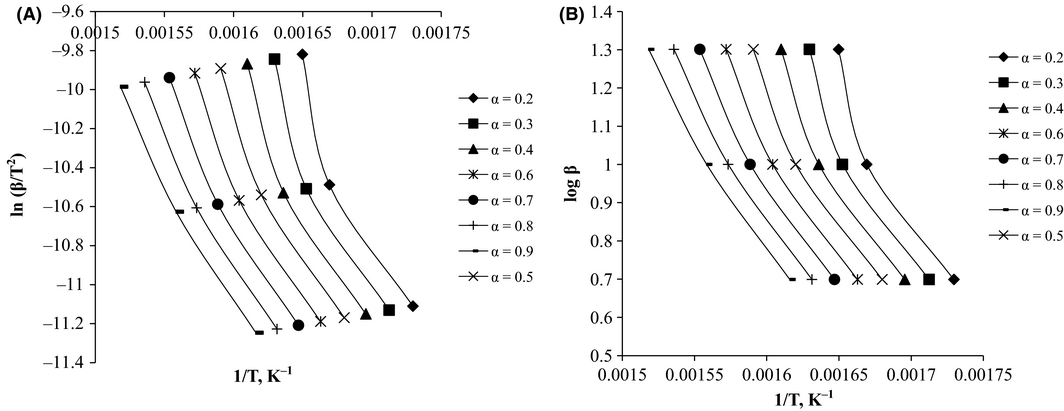Figure 3. Regression lines for the determination of activation energy by (A) Kissinger–Akahira–Sunose (KAS) and (B) Ozawa–Flynn–Wall (OFW) methods.

Activation energies, calculated from the KAS and OFW methods, are presented in Table 3. It is evident from the table that the values obtained employing both methods are similar. Regression coefficients of the plots increased with progressive conversions. Activation energies from KAS method ranged from 122.8 kJ/mol to 106 kJ/mol for conversions ranging from α = 0.2 to α = 0.9. The activation energies from the OFW method were in the range 126–110 kJ/mol for the same conversions.

Table 3. Arrhenius parameters and regression factors at different conversions from KAS and OFW methods
Conversion, α KAS method OFW method A, min−1
Ea, kJ/mol R2 Ea, kJ/mol R2
1. KAS, Kissinger–Akahira–Sunose method; OFW, Ozawa–Flynn–Wall method.

0.2 122.81 0.907 126.03 0.919 1.75 × 1010
0.3 120.41 0.927 123.87 0.937 1.54 × 1010
0.4 117.92 0.943 121.61 0.951 1.28 × 1010
0.5 114.26 0.958 117.95 0.962 8.11 × 109
0.6 112.97 0.967 117.10 0.972 9.23 × 109
0.7 108.24 0.975 114.92 0.979 9.08 × 109
0.8 108.24 0.982 112.83 0.985 1.15 × 1010
0.9 106.00 0.987 110.81 0.989 2.54 × 1010

Activation energies decreased with an increase in conversion, as high conversions were observed at high temperatures, due to the requirement of comparatively lower activation energies at elevated temperatures. More reactions were triggered at higher temperatures, leading to a sharp rise in reaction rates with more unstable intermediates and lower activation energies. The reported values of pyrolysis reaction activation energies for hazelnut husk are in the range 128–131 kJ/mol , 129 kJ/mol for corn cob , and 62–206 kJ/mol for pine wood waste ; the activation energy of pyrolysis of karanja seed cake are in the range of the hazelnut husk and corn cob, but there was considerable deviation from the reported activation energy of pine wood biomass. The activation energy of pyrolysis is highly dependent on the extent of conversion and the type and composition of biomass feedstock as seen in the activation energy for pine wood waste which is a woody biomass, rich in lignin, and very different from that of seed cake biomass and nut husk and other biomass types. KAS and OFW methods gave reliable activation energies that were used for the calculation of the pre-exponential factor in order to get a complete picture of the kinetic parameters with progressive conversions, the pre-exponential factors were determined by making use of the reaction model obtained from Coats-Redfern (CRF) method. Calculated pre-exponential factor values from OFW method are shown in Table 3. Therefore, the kinetic expression obtained for pyrolysis of karanja seed cake was:

 ${\frac {d\alpha }{dt}}=Ae^{-{\frac {E}{RT}}}{\left(1-\alpha \right)}^{3}$
(13)

where the Arrhenius parameters obtained from model-free isoconversional methods were in good agreement with the values determined using model-fitting method.

Obtaining of the pyrolysis kinetic parameters based on TGA experimental data is extremely useful in the design and control of pyrolysis process especially large scale operation with desired composition and yield of products. Moreover, pyrolysis kinetic data link technological parameters, importantly biomass hold up time at preset temperature program that determines pyrolysis products profile.

### Characterization of thermally treated biomass

Thermal treatment of karanja seed cake was performed in a fixed bed reactor of 1 cm inner diameter and 35 cm length under slow pyrolysis conditions with a continuous supply of nitrogen gas at 0.1 liter per minute (LPM) flow at different temperatures including 200, 250, 300, 350, 400, 450, and 500°C. The pyrolysis transformed the karanja seed cake to biochar (thermally treated biomass retained in the reactor), bio-oil (condensed liquid product), and the gases. The solid and the liquid obtained were weighed and the yields of the same were expressed gravimetrically. Biochar was characterized by proximate analysis carried out according to ASTM standard methods  using Mettler Toledo TGA/SDTA 851e analyzer (Switzerland), ultimate/elemental analysis using a CHNS Analyzer–ELEMENTARVario microcube model, surface structure was obtained by scanning electron microscopy (SEM) using Hitachi S-3000N scanning electron microscope, Japan and FTIR (Fourier transform infrared spectroscopy) using a Perkin Elmer System 100 Fourier transform infrared spectrometer (Perkin Elmer Ltd., Seer Green, Beaconsfield, Bucks HP9 2FX, United Kingdom) .

Pyrolysis temperature showed a profound influence on the product distribution in the reaction. Figure 4 shows the pyrolysis products, biochar, and total volatiles and yields obtained in relation to temperature. Pyrolysis at higher temperature (≥500°C) resulted in increased carbon conversion into volatiles [27, 28] and reduced biochar.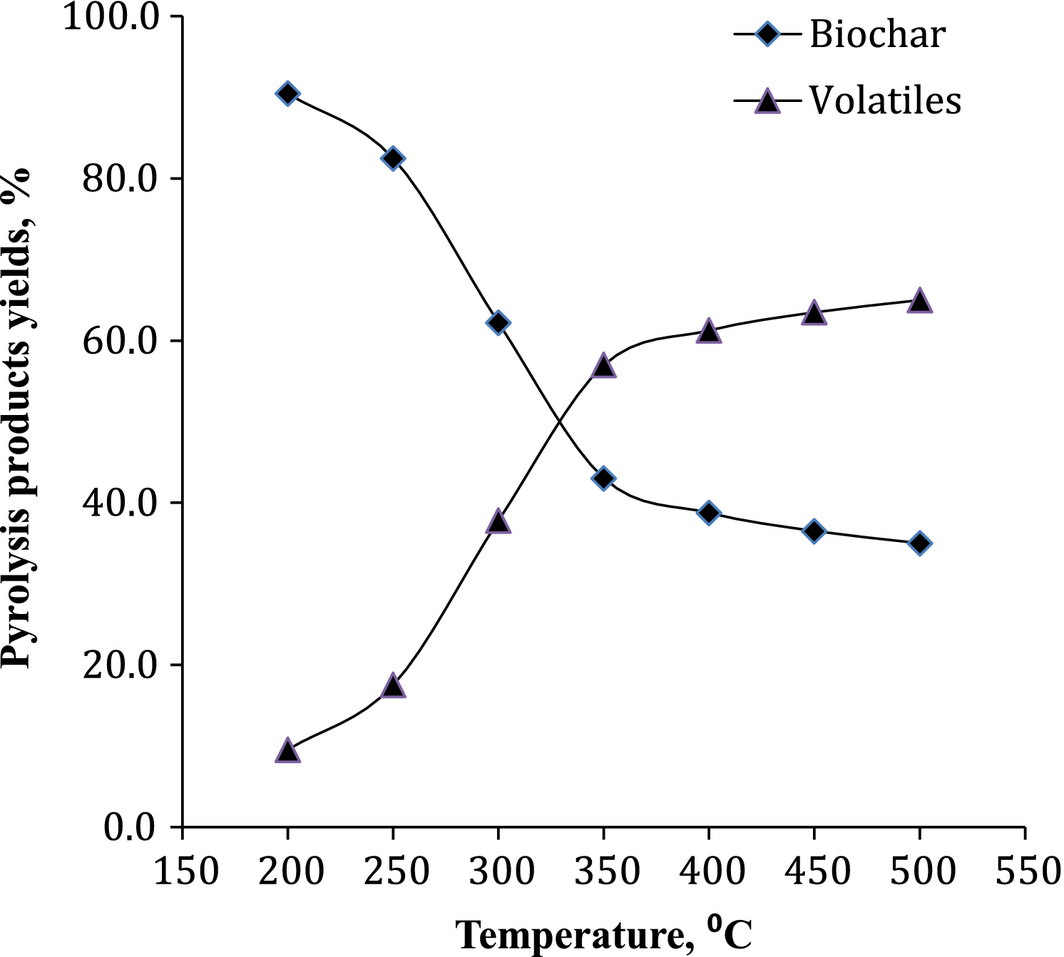Figure 4. Effect of temperature on biochar and total volatiles yield from pyrolysis.

Karanja seed cake, processed in the present work, composed of 25.2% carbohydrates, 17.4% lignin, 13.4% protein, and 4% ash with 40% water and ethanol soluble matter, details of which were presented in our earlier work . Biochar obtained from the thermal treatment of karanja seed cake was analyzed for its composition in terms of proximate and ultimate analysis and the data were presented in Table 4 along with evaluated HHV, carbon carryover, H/C and O/C ratios. From the elemental analysis of feed karanja seed cake and biochar at different temperatures it was observed that the carbon content increased, whereas the hydrogen and oxygen contents decreased with increase in temperature. H/C and O/C values give a picture of the degree of carbonization, aromaticity, and maturity of organic materials. A decrease in oxygen (O/C) with increase in temperature was due to the increased decomposition of oxygen containing functional groups. The percent biochar obtained from the seed cake by experimentation was 56.82%, 53.29%, 52.55%, and 51.52% at 350, 400, 450, and 500°C, respectively, indicating a decrease in the carbon carryover from the seed cake to biochar with temperature. Therefore, the value addition of deoiled karanja seed cake by thermal treatment is a promising pretreatment method to obtain biochar for multiple applications.

Table 4. Composition and energy value of biochar obtained from pyrolysis of karanja seed cake at different temperatures
Element, %wt. Karanja cake Biochar from pyrolysis at
350°C 400°C 450°C 500°C
1. a

(Carbon content in biochar × Biochar yield)/(Carbon content in karanja seed cake).

Proximate analysis
Moisture 06.7       03.20
Ash 04.00       15.42
Volatile matter 71.44       14.13
Fixed carbon 17.86       67.25
Ultimate analysis
N 04.30 05.84 05.91 06.07 05.95
C 44.20 58.39 60.80 63.64 65.06
H 06.50 05.21 04.45 04.61 04.30
S
O 45.00 30.56 28.84 25.68 24.69
HHV, MJ/kg 18.11 22.9 23.14 24.23 24.5
H/C 1.76 1.07 0.91 0.83 0.80
O/C 0.68 0.35 0.31 0.27 0.26
Carbon carryovera, % 56.82 53.29 52.55 51.52

#### Scanning electron microscopy

As porosity play a vital role in determining the efficiency of biosorbents in the adsorption of contaminants in effluent treatment, with maximum adsorption occurring when pores are large enough to admit contaminant molecules, SEM of karanja cake and biochar was carried out to assess the surface structure of the samples. The SEM pictures obtained are presented in Figure 5A and B. As is evident from the pictures, the char formed (Fig. 5B) has a highly porous structure compared to the original seed cake biomass (Fig. 5A). This can be attributed to the fact that thermal treatment improves the porous structure of biochar due to the loss of hydrogen, oxygen (major quantity), and carbon (minor quantity) atoms in the form of volatiles from the parent biomass material leaving the skeletal structure.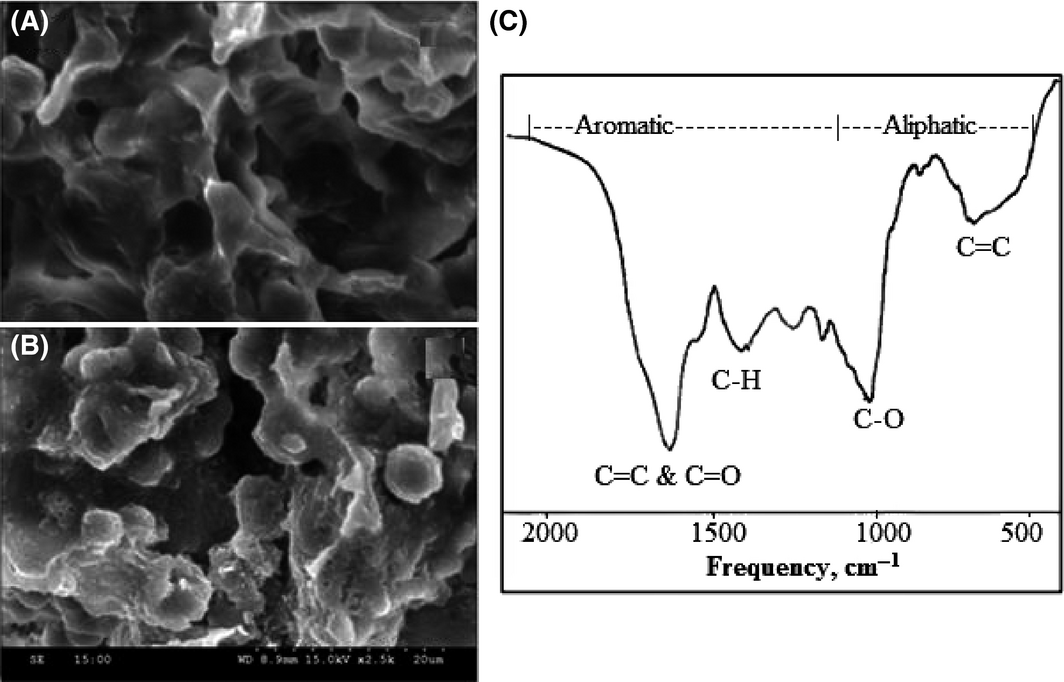Figure 5. Surface structure (from scanning electron microscopy [SEM]) of (A) karanja seed cake biomass and (B) biochar from karanja seed cake, and (C) Fourier transform infrared spectra of biochar obtained at 500°C.

#### Fourier transform infrared spectroscopy

Fourier transform infrared spectra of biochar was collected using the KBr pelletization method on a Perkin Elmer System 100 Fourier transform infrared spectrometer (FTIR) with a cesium iodide (CsI) micro-focus accessory and triglycine sulfate (TGS) detector by scanning from 4000 to 400 cm−1, with a resolution of 4 cm−1. The biochar spectra as shown in Figure 5C depicts the vibrations in the frequency range 700–1200 cm−1 and 1200–2000 cm−1 confirming the aliphatic and aromatic nature of the sample. The peaks at frequencies of 698 (aromatic ring C-H), 1020 (C-O stretching vibration), 1420 (C-H stretching vibration), and 1626 cm−1 (C=C and C=O stretching vibrations) show the carbon structure of biochar. This spectrum is comparable to reference spectra obtained by Rutherford et al.  who studied changes in composition and porosity occurring during the thermal degradation of wood and wood components. The result further emphasizes the fact that thermal treatment of biomass converts aliphatic carbon to aromatic on prolonged treatment .

From the characterization of the seed cake biomass and biochar based on the elemental composition, surface structure, and FTIR, it is evident that the biochar is rich in carbon and has porous structure and can be effectively used for energy generation and as biosorbent for industrial effluent treatment and electrode material in activated carbon electrodes manufacture.

## Conclusions

This study is the first report on nonedible seed cake biomass pyrolysis kinetics in particular karanja seed cake biomass, and the work presented is extremely helpful to understand the usefulness of value addition to karanja seed cake through thermal treatment. The TGA pyrolysis reactions at constant heating rate revealed that a temperature of 450–500°C was sufficient for carbonization of the biomass. The KAS, OFW, and CRF methods gave reliable activation energies and pre-exponential factors with third-order reaction model for the seed cake pyrolysis reaction. Characterization results showed that the thermally treated biomass has high porous structure and rich in carbon, suitable for various applications as biosorbent and precursor for carbon materials. The kinetic data thus obtained are extremely useful in design of pyrolysis system for different seed cake biomass.

## Conflict of Interest

None declared.

### Document informationPublished on 01/06/17
Submitted on 01/06/17

Licence: Other

### Document Score0

Views 73
Recommendations 0